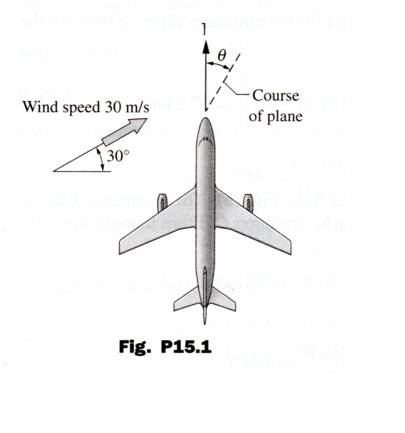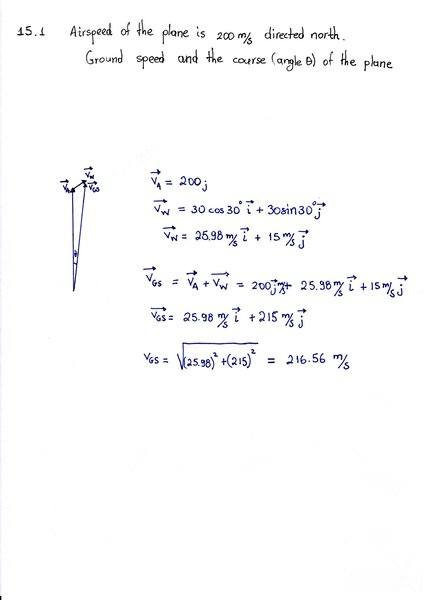# Determine the ground speed and the course of the plane

• Alexanddros81

## Homework Statement

15.1 The airspeed of the plane is 200m/s, directed north. If the wind speed is 30m/s
in the direction shown, determine the ground speed and the course (angle θ) of the plane.

## Homework Equations## The Attempt at a SolutionIs it so far correct the above?
More questions will follow regarding the vector diagram and understanding of theory

#### Attachments

It seems correct, but you do not really need to compute the components. Just apply the cosine and sine theorems on the vector diagram.